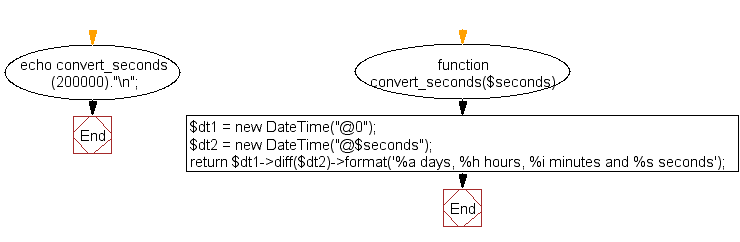﻿ PHP Date Exercise: Convert seconds into days, hours, minutes and seconds - w3resource# PHP Date Exercises : Convert seconds into days, hours, minutes and seconds

## PHP date: Exercise-21 with Solution

Write a PHP script to convert seconds into days, hours, minutes and seconds.

Sample seconds : 200000

Sample Solution:

PHP Code:

``````<?php
function convert_seconds(\$seconds)
{
\$dt1 = new DateTime("@0");
\$dt2 = new DateTime("@\$seconds");
return \$dt1->diff(\$dt2)->format('%a days, %h hours, %i minutes and %s seconds');
}
echo convert_seconds(200000)."\n";
?>
```
```

Sample Output:

```2 days, 7 hours, 33 minutes and 20 seconds
```

Flowchart :PHP Code Editor:

Have another way to solve this solution? Contribute your code (and comments) through Disqus.

What is the difficulty level of this exercise?

﻿

## PHP: Tips of the Day

SQL injection that gets around mysql_real_escape_string()

Consider the following query:

```\$iId = mysql_real_escape_string("1 OR 1=1");
\$sSql = "SELECT * FROM table WHERE id = \$iId";
```

mysql_real_escape_string() will not protect you against this. The fact that you use single quotes (' ') around your variables inside your query is what protects you against this. The following is also an option:

```\$iId = (int)"1 OR 1=1";
\$sSql = "SELECT * FROM table WHERE id = \$iId";
```

Ref : https://bit.ly/32q3bJ7